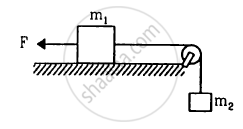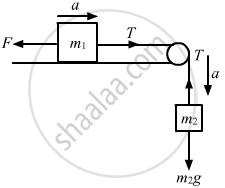Department of Pre-University Education, KarnatakaPUC Karnataka Science Class 11
Advertisement Remove all ads

# Consider the situation shown in figure. The force F is equal to the m2g/2. If the area of cross section of the string is A and its Young modulus Y, find the strain developed in it. - Physics

Answer in Brief

Consider the situation shown in figure. The force F is equal to the m2 g/2. If the area of cross section of the string is A and its Young modulus Y, find the strain developed in it. The string is light and there is no friction anywhere.Advertisement Remove all ads

#### Solution

Given:
Force (F) = m2g/2
Area of cross-section of the string = A
Young's modulus = Y
Let a be the acceleration produced in block m2 in the downward direction and T be the tension in the string.
From the free body diagram:

$\text{m}_2 \text{g - T = m}_2 \text{a} . . . \left( \text{i} \right)$
$\text{ T - F = m}_1 \text{a . . . (ii)}$From equations (i) and (ii), we get:

$a = \frac{\text{m}_2 \text{g - F}}{\text{m}_1 + \text{m}_2}$
$\text{ Applying F }= \frac{\text{m}_2 \text{g}}{2}$
$\Rightarrow a = \frac{\text{m}_2 \text{g}}{2\left( \text{m}_1 + \text{m}_2 \right)}$

Again, T = F + m1a

On applying the values of F and a, we get:

$\Rightarrow T = \frac{\text{m}_2 \text{g}}{2} + \text{m}_1 \frac{\text{m}_2 \text{g}}{2\left( \text{m}_1 + \text{m}_2 \right)}$
We know that:
$\text{Y} = \frac{\text{FL}}{\text{A ∆ L}}$
$\Rightarrow \text{ Strain }= \frac{∆ \text{L}}{\text{L}} = \frac{\text{F}}{\text{AY}}$
$\Rightarrow \text{ Strain } = \frac{\left( \text{m}_2^2 + 2 \text{m}_1 \text{m}_2 \right)\text{g}}{2\left( \text{m}_1 + \text{m}_2 \right) \text{AY}}$
$= \frac{\text{m}_2 g\left( 2 \text{m}_1 + \text{m}_2 \right)}{2\text{AY} \left( \text{m}_1 + \text{m}_2 \right)}$
∴ Required strain developed in the string $= \frac{\text{m}_2 g\left( 2 \text{m}_1 + \text{m}_2 \right)}{2\text{AY} \left( \text{m}_1 + \text{m}_2 \right)}$ .
Is there an error in this question or solution?
Advertisement Remove all ads

#### APPEARS IN

HC Verma Class 11, 12 Concepts of Physics 1
Chapter 14 Some Mechanical Properties of Matter
Q 9 | Page 300
Advertisement Remove all ads
Advertisement Remove all ads
Share
Notifications

View all notifications

Forgot password?